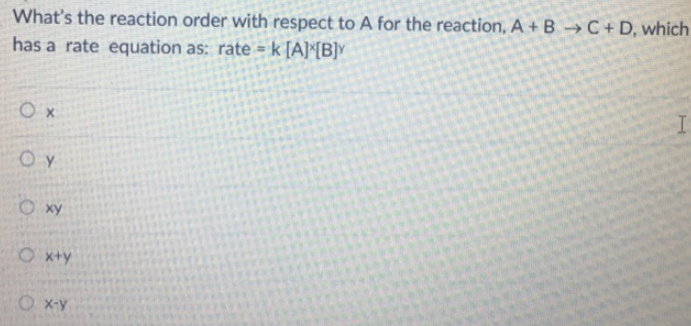# Problem: What's the reaction order with respect to A for the reaction, A + B → C + D, which has a rate equation as: rate = k[A]x[B]y(a) x(b) y(c) xy(d) x+y(e) x-y

###### FREE Expert Solution
100% (473 ratings)###### Problem Details

What's the reaction order with respect to A for the reaction, A + B → C + D, which has a rate equation as: rate = k[A]x[B]y

(a) x

(b) y

(c) xy

(d) x+y

(e) x-y